R provides a number of functions associated with commonly used probability distributions. For each probability distribution there are four functions associated with it. They carry the prefixes d, p, q and r. The prefix d stands for “density”, returning the probability density function; p means “probability”, returning the cumulative distribution function; q means “quantile”, returning the inverse of the cumulative distribution function; r means “random”, returning random numbers following the specified probability distribution.

## Normal Distribution

In this section we introduce the R functions associated with the most commonly used probability distribution in Statistics: the normal distribution. The associated R functions are dnorm(), pnorm(), qnorm() and rnorm(). Type ?dnorm in the R console for the detail of their usage.

### dnorm()

The dnorm(x) function returns the density of the standard normal distribution (mean=0, standard deviation=1) at x. The mathematical function for the distribution is $P(x) = \frac{1}{\sqrt{2\pi}}e^{-x^2/2}$ For example,

dnorm(-3:3)
 0.004431848 0.053990967 0.241970725 0.398942280 0.241970725 0.053990967
 0.004431848

returns the density $$P(x)$$ at $$x$$ = -3, -2, -1, 0, 1, 2 and 3. We see that the distribution is symmetric about $$x = 0$$. The standard normal curve can be plotted using the command

curve(dnorm(x), xlim=c(-3,3))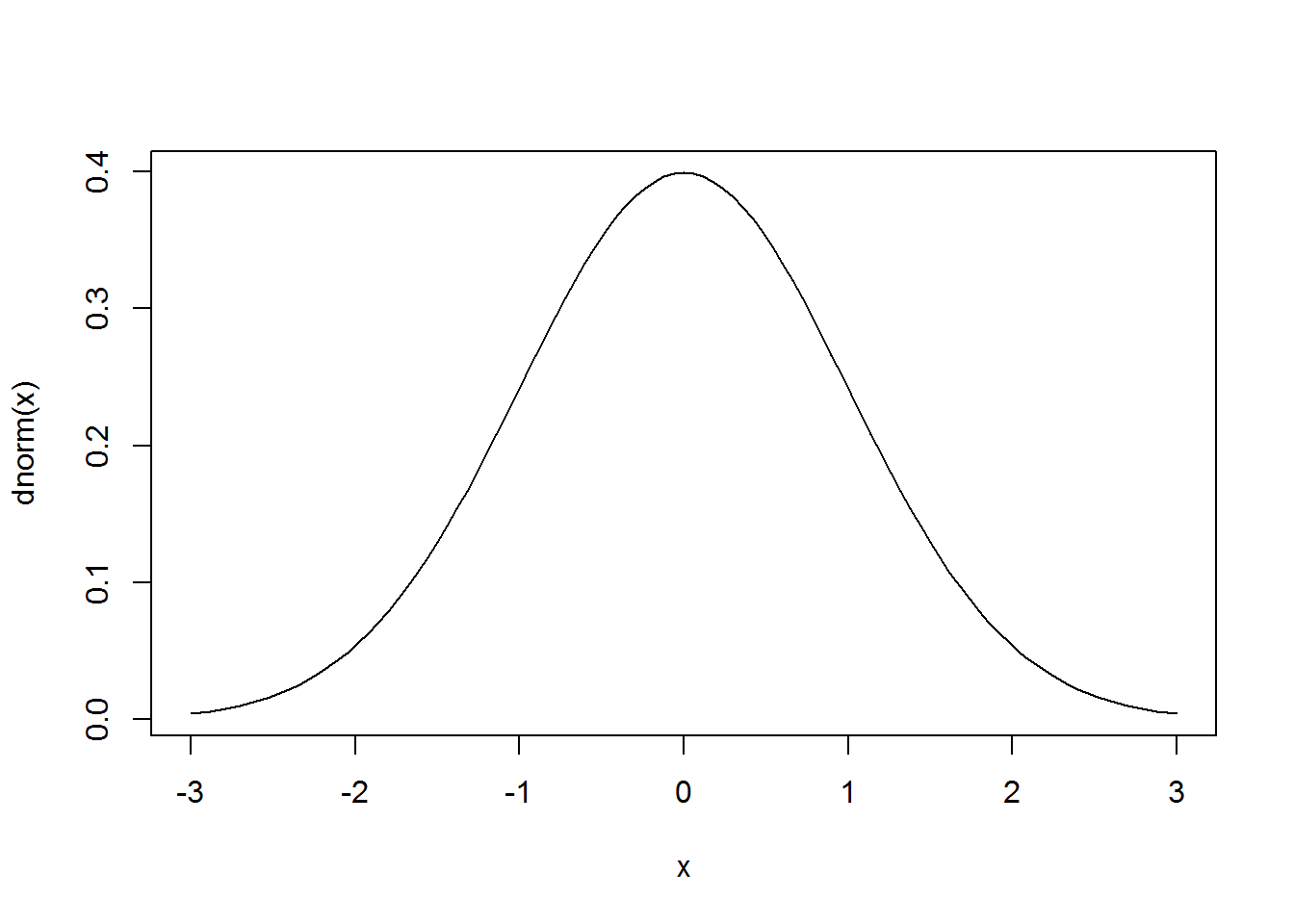The xlim=c(-3,3) tells R to plot the function in the range $$-3 \leq x \leq 3$$. Type ?curve to see other available options for the curve() function.

The dnorm() function has other options that allow you to choose normal distributions with another mean and standard deviation (again type ?dnorm to see the usage). The mathematical equation for the distribution is $P(x;\mu,\sigma)=\frac{1}{\sqrt{2\pi \sigma^2}}e^{-(x-\mu)^2/(2\sigma^2)}$ Here $$\mu$$ is the mean and $$\sigma$$ is the standard deviation. For example, the following commands plot the standard normal curve, $$P(x)$$ (black) and a normal curve with mean = 1 and standard deviation = 2, $$P(x;1,2)$$ (red):

curve(dnorm(x), xlim=c(-6,6))
curve(dnorm(x, mean=1, sd=2), col="red", add=TRUE)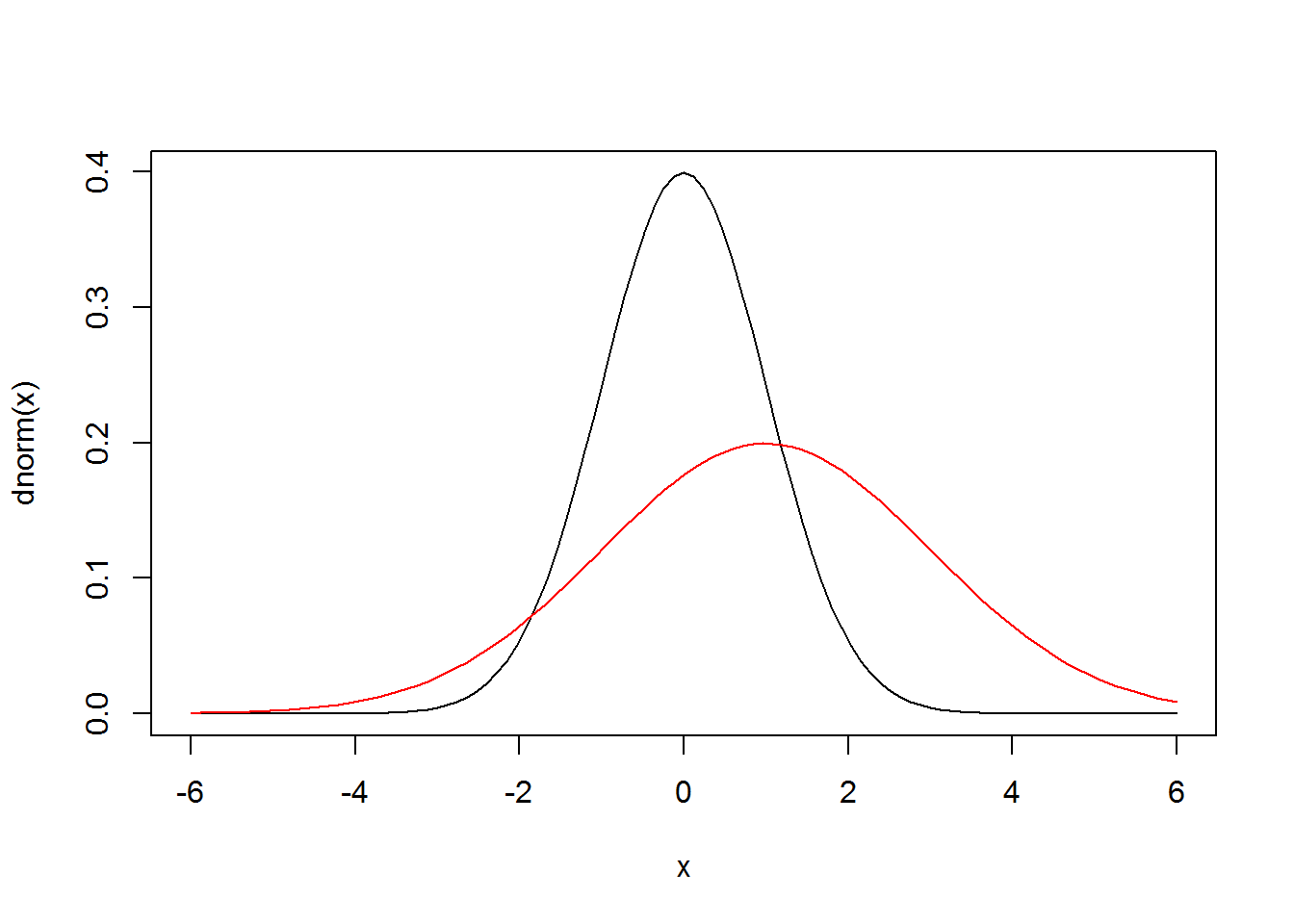The first line in the code chunk plots the standard normal curve in the range $$-6 \leq x \leq 6$$. In the second line, col=“red” plots the function with a red line. The add=TRUE means that the plot should be added to the first plot instead of generating a new plot. We see that the second curve is symmetric about $$x=1$$ and is more spread out compared to the standard normal curve.

### pnorm() and qnorm()

The pnorm(z) function returns the cumulative probability of the standard normal distribution at Z score $$z$$. That is, it’s the area under the standard normal curve to the left of $$z$$ (the area of the shaded blue region in the plot below).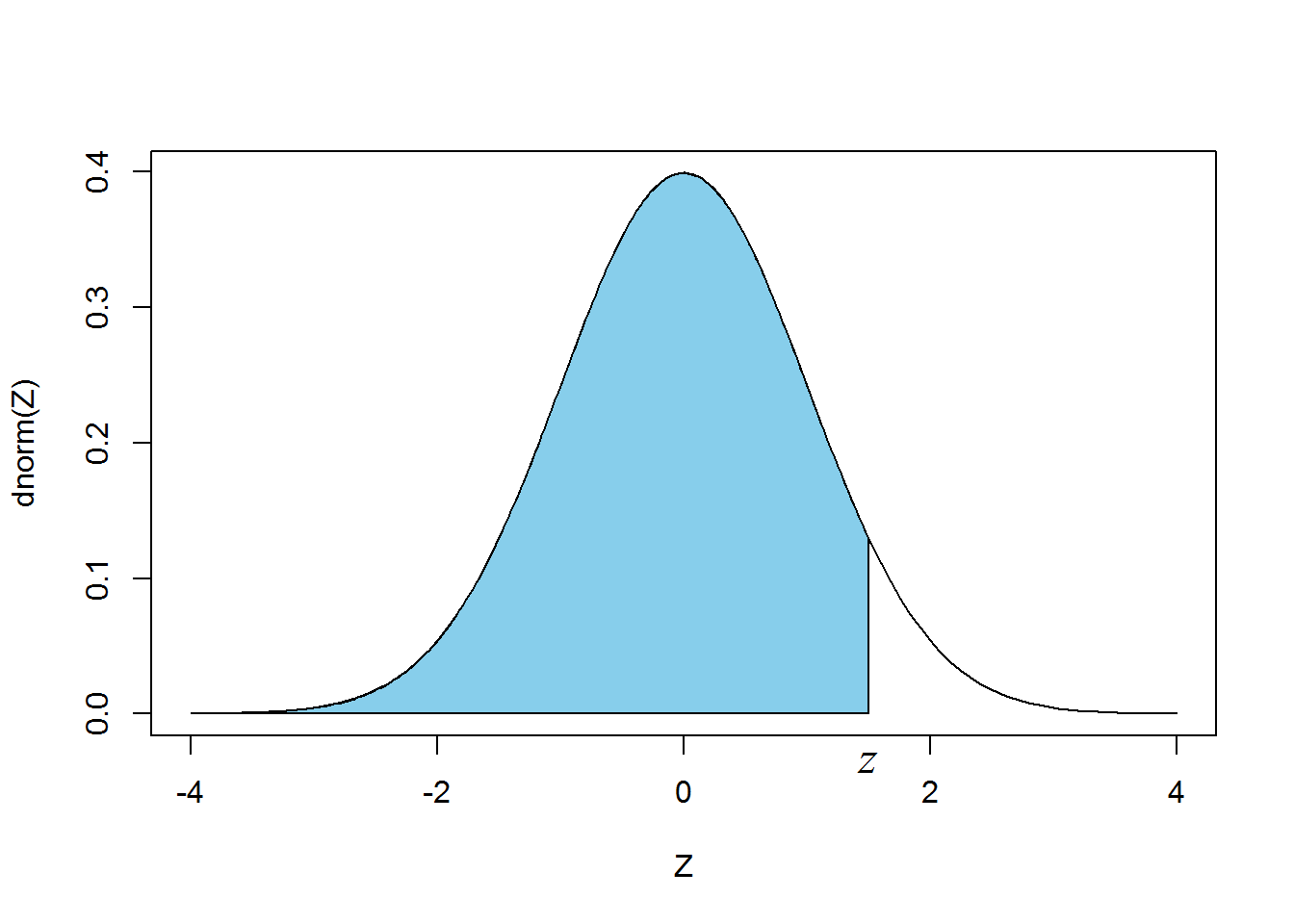For example,

pnorm(1.65)
 0.9505285

This means that the probability of getting a Z score smaller than 1.65 is 0.95 or 95%. Sometimes, we want to find the area of the right-side tail (area of the shaded region below).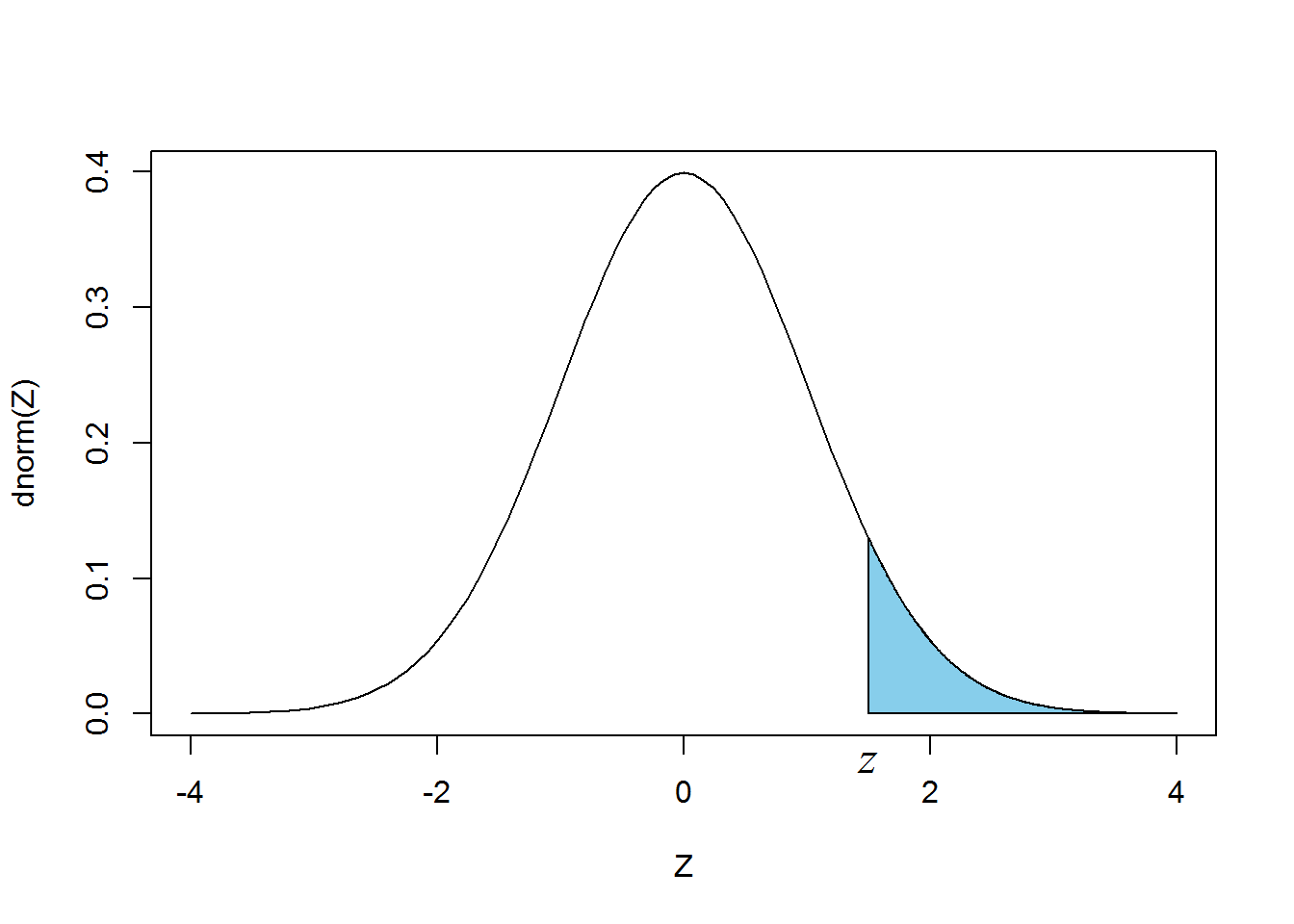This is especially the case when we want to find the (one-sided) p-value corresponding to a Z score. There are many ways to do this. The first method is to use the fact that the normal curve is symmetric, so $$P(Z>z)=P(Z<-z)$$. For example, $$P(Z>1.65)=P(Z<-1.65)$$ and $$P(Z>1.65)$$ can be computed by

pnorm(-1.65)
 0.04947147

The second method is to use $$P(Z>1.65)=1-P(Z<1.65)$$ and $$P(Z>1.65)$$ can be computed by

1-pnorm(1.65)
 0.04947147

The third method is to the option lower.tail=FALSE in pnorm():

pnorm(1.65,lower.tail=FALSE)
 0.04947147

We see that the three methods produce the same result, as expected. I prefer the first method as it involves the least typing.

The qnorm() function is the inverse of the pnorm() function: qnorm(y) returns the value $$x$$ so that pnorm(x)=y. For example, the Z score corresponding to the 95th percentile is

qnorm(0.95)
 1.644854

We can check that the result is correct:

pnorm( qnorm(0.95) )
 0.95

Like the pnorm() function, the option lower.tail=FALSE refers to the area of the right tail. For example,

qnorm(0.05,lower.tail=FALSE)
 1.644854

This means that the Z score corresponding to the area of the right tail equaling 0.05 is 1.645. It can also be obtained by the following expression because of the symmetry of the normal curve (the Z score is the negative of the Z score corresponding to the area of the left tail being 0.05):

-qnorm(0.05)
 1.644854

### rnorm()

The rnorm() function is a random number generator (type ?rnorm to see its usage). For example,

rnorm(10)
   0.4053546 -0.6914374 -0.5623105  0.2652098 -0.9166066  0.6804066
 -0.7471598 -1.1550152 -0.6181327  0.7914630

generates 10 random numbers following the standard normal distribution. It returns different numbers the next time you run it:

rnorm(10)
   2.4412312  1.2042262  0.5926276  0.3633825 -1.9873193  0.5377273
 -0.9754285 -0.9924121 -1.4767626 -0.9644406

The command

rnorm(20,mean=1,sd=3)
   7.4726921 -4.1817994  1.4767907 -0.9934669  3.2575528  0.4522079
  1.7966542 -3.3782191  1.9933302  0.9305993 -1.2038704  0.8175358
 -1.5344569  3.9262773  0.2872347  3.0199772 -1.6123880  3.0520579
  0.2618046 -2.6131915

generates 20 random numbers following the normal distribution with mean = 1 and standard deviation = 3. All random numbers generated by computers are not truly random, in the sense that these numbers are generated based on some rules. They appear to be random and have many of the statistical properties of true random numbers. For this reason they are often called the pseudo random numbers. Pseudo random numbers are adequate in most statistical applications.

In many applications, we want to be able to reproduce the results of our data analysis. It is then important to use the set.seed(n) function to generate the same set of random numbers. Here $$n$$ is an integer. For example, the commands

set.seed(107281)
rnorm(10)
   0.12977398 -0.93544505  0.44900614 -0.85894095  0.03539138
 -0.31035867 -1.01223948 -0.70036977 -0.92594897 -0.53929791

sets a seed (107281) and then generates 10 standard normal random numbers. When we run rnorm(10) again, we get different numebrs:

rnorm(10)
   0.81412055 -0.65041261  0.76975912  1.46504444 -0.07391547
 -0.28979138 -1.43520277 -0.20861926 -0.44830295 -0.58084481

However, when we reset the seed to 107281 and generate 20 standard normal random numbers,

set.seed(107281)
rnorm(20)
   0.12977398 -0.93544505  0.44900614 -0.85894095  0.03539138
 -0.31035867 -1.01223948 -0.70036977 -0.92594897 -0.53929791
  0.81412055 -0.65041261  0.76975912  1.46504444 -0.07391547
 -0.28979138 -1.43520277 -0.20861926 -0.44830295 -0.58084481

we get the exact same 20 numbers as before. You will see that I always use set.seed() before generating a new set of random numbers. This is mainly for the purpose of reproducibility. In general, it is a good idea to use set.seed() if you are working on a project that requires the use of random numbers, at least at the beginning. This is especially useful if the project involves complicated coding as the reproducibility makes the code debugging easier.

Random numbers are essential in statistical simulations and we will talk more about them later in the course.

Now let’s study some properties of the random number generated by the rnorm() function. The following code chunk generates 5 sets of standard normal random numbers.

set.seed(17289143)
# Generate 5 sets of standard normal random nums with length=1000
x1 <- rnorm(1000)
x2 <- rnorm(1000)
x3 <- rnorm(1000)
x4 <- rnorm(1000)
x5 <- rnorm(1000)

The # symbol is a comment character. R ignores everything following # in the rest of a line. One important property of random numbers is that they are independent, and so are uncorrelated. Let’s check to see if the 5 sets of random numbers are uncorrelated by computing the correlation matrix:

cor(cbind(x1,x2,x3,x4,x5))
             x1           x2           x3           x4          x5
x1  1.000000000 -0.002318008  0.017276589  0.081279622 0.042118493
x2 -0.002318008  1.000000000  0.002570233 -0.010809180 0.042593306
x3  0.017276589  0.002570233  1.000000000 -0.020561573 0.018972720
x4  0.081279622 -0.010809180 -0.020561573  1.000000000 0.001248967
x5  0.042118493  0.042593306  0.018972720  0.001248967 1.000000000

We see that the off-diagonal elements of the matrix are small, indicating that they are uncorrelated.

Now let’s generate $$10^5$$ standard normal random numbers to analyze their statistical properties.

set.seed(2819237)
x <- rnorm(1e5)

We can look at the mean and standard deviation:

mean(x)
 -0.00424089
sd(x)
 0.999141

Note that I don’t bother to multiply the sd by $$\sqrt{(N-1)/N}$$ since $$N=10^5$$ is very large and $$\sqrt{(N-1)/N}$$ is very close to 1. We see that the mean and sd are very close to 0 and 1, respectively, as expected. To verify that the data is normally distributed, we generate a histogram using the hist() function:

hist(x)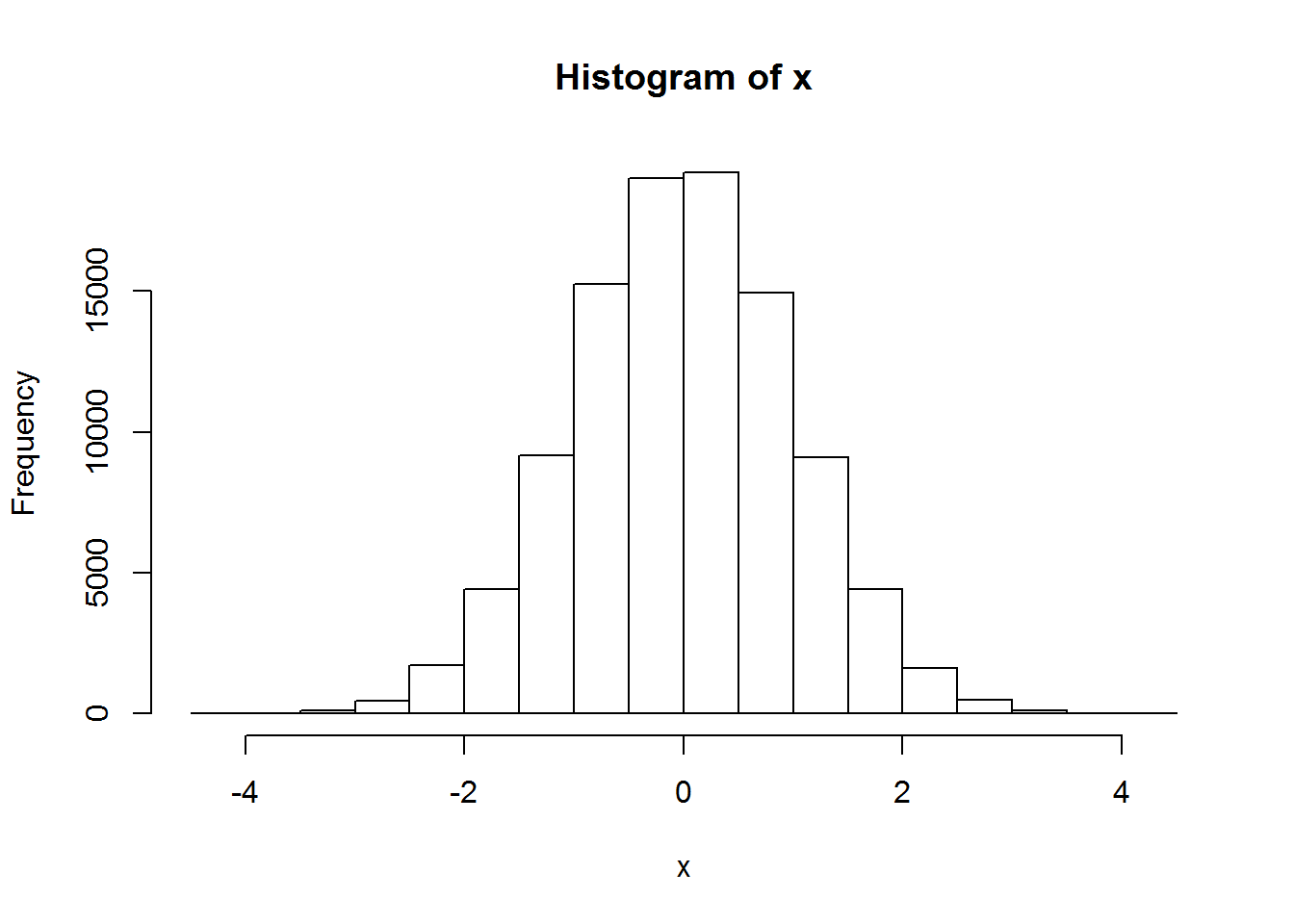The histogram looks like a normal curve, but we should be able to do a better job by breaking the histogram into smaller intervals. Like many other R functions, there are parameters in the hist() function that allow us to customize our histogram. As you are diving into the R world, you will encounter many R functions having many optional parameters. It is important to note that you don’t need to memorize all the parameters as you can easily find them by pulling up a help page using ?. If you don’t understand the help page, do an internet search and you will be amazed to find that many webpages have the information you are looking for.

Here is a better histogram for our data:

hist(x, freq=FALSE, breaks=100)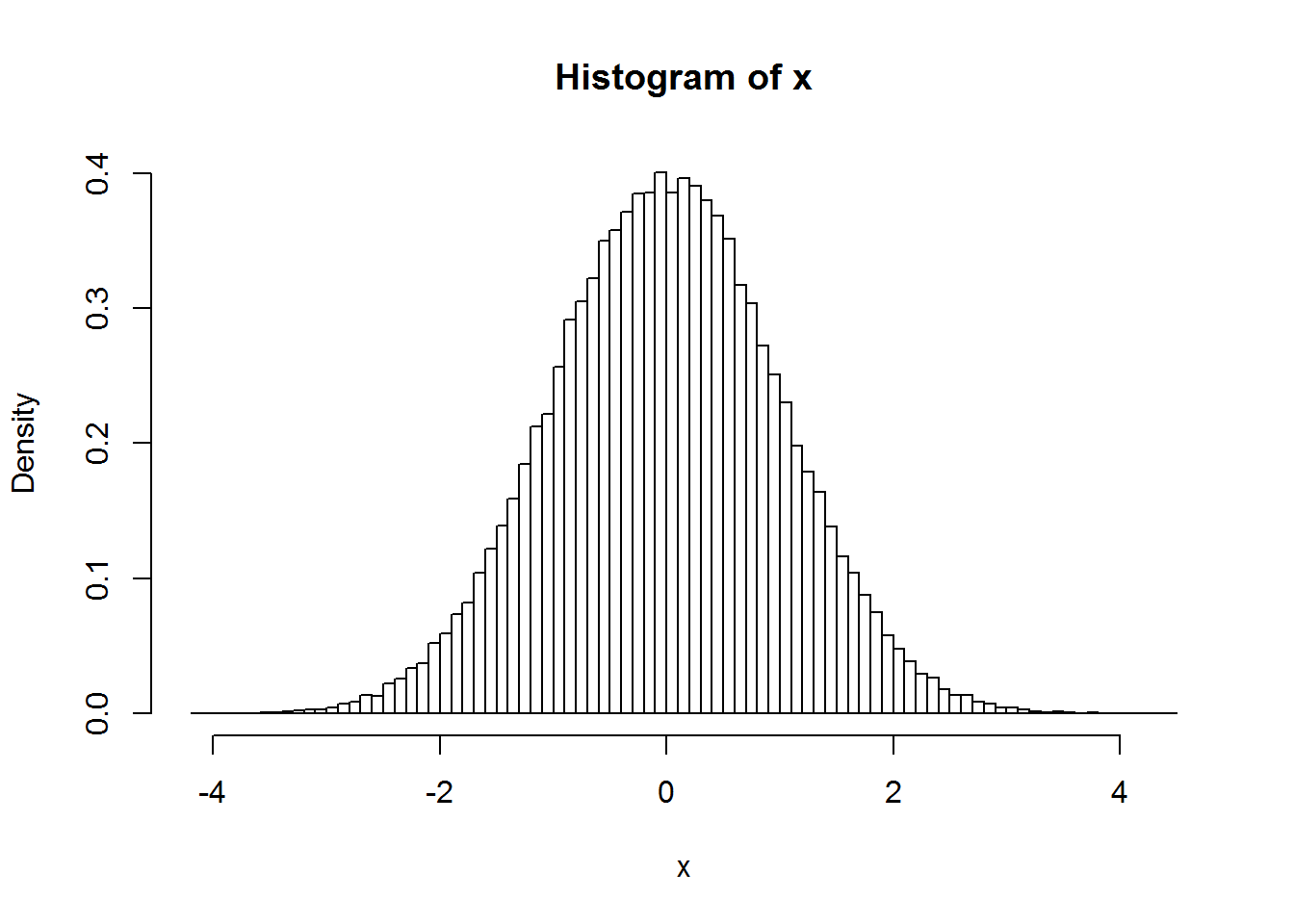The freq=FALSE option is to show the density (fraction of points in each interval) on the y-axis instead of frequency (number of points in each interval). With this option, the total area of the histogram is normalized to 1. The breaks=100 option is to draw the histogram with 100 equally spaced intervals. This histogram looks more like the standard normal curve. In fact, we can plot the standard normal curve on top of the histogram to have a better comparison:

hist(x, freq=FALSE, breaks=100)
curve(dnorm(x),col="red",add=TRUE)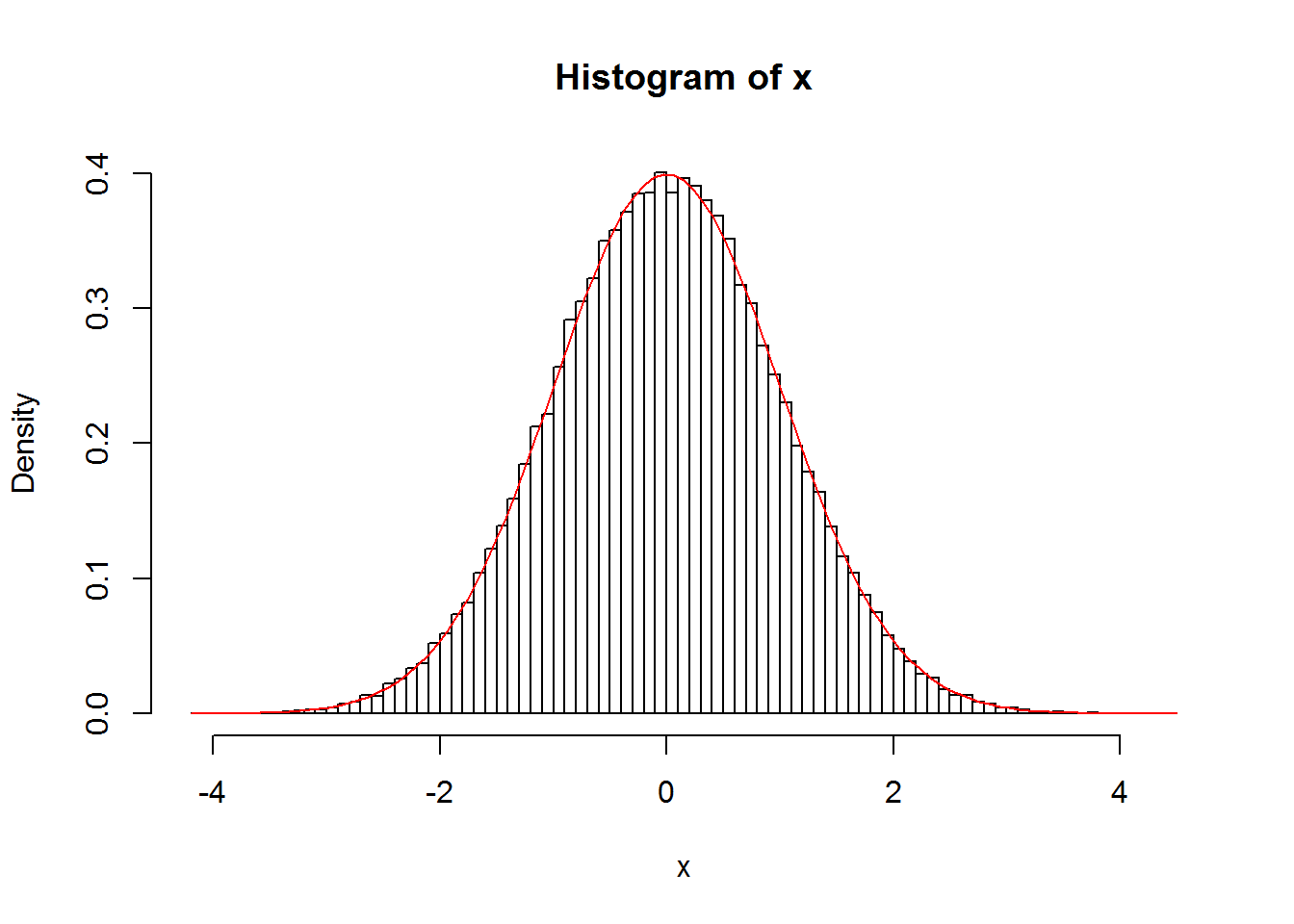We thus verify that the rnorm() function generates random numbers following the normal distribution. Let’s try something new.

Exercise 1: Make a histogram of $$x^2$$. Does it resemble anything you are familiar with?

## $$\chi^2$$ Distribution

The four functions associated with the $$\chi^2$$ distribution are dchisq(), pchisq(), qchisq(), rchisq(). The usage is essentially the same as the four functions associated with the normal distribution. Again, use ?dchisq to find out more information.

Before we demonstrate the use of these four functions, let’s first talk about what $$\chi^2$$ distribution is. A $$\chi^2$$ distribution with $$k$$ degrees of freedom is constructed by the sum of the square of $$k$$ independent, standard normal random variables. Specifically, if $$X_1$$, $$X_2$$, $$\cdots$$, $$X_k$$ are independent random variables and they all follow the standard normal distribution, then the random variable $Q = X_1^2+ X_2^2 + \cdots + X_k^2$ follows the $$\chi^2$$ distribution with $$k$$ degrees of freedom. If you find the concept hard to follow, read this additional note on random numbers.

### dchisq() and rchisq()

The mathematical functions for the $$\chi^2$$ density distribution can be found in many standard textbooks and from the internet (e.g., Wikipedia). To see what the $$\chi^2$$ distributions look like, we use the dchisq() function to plot the density functions:

curve(dchisq(x,1),xlim=c(0,15),ylim=c(0,0.6),ylab="Chi Square Density")
legend(12,0.55,c("k=1","k=2","k=3","k=5","k=10"),
col=c("black","red","blue","dark green","brown"),lty=1:5)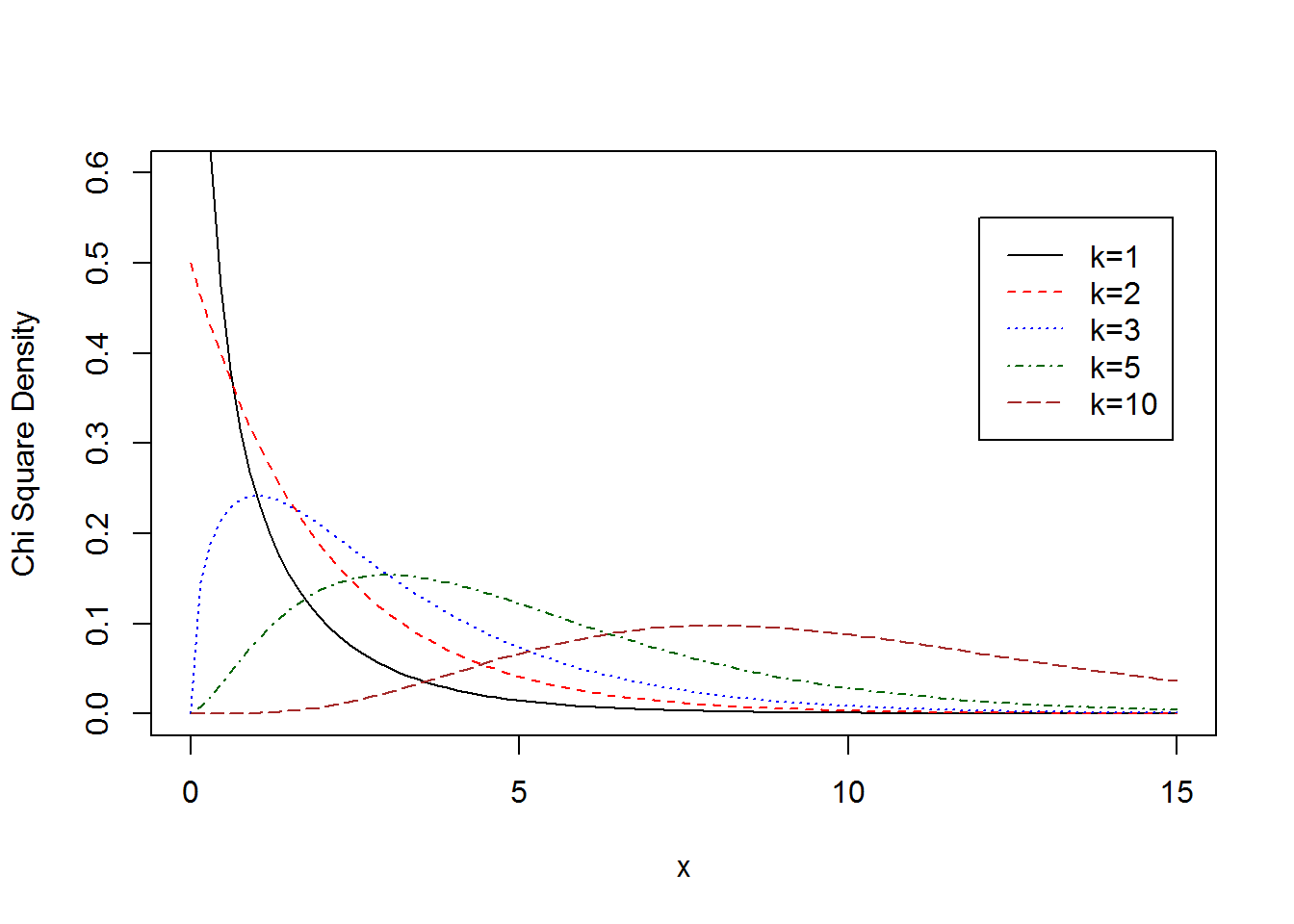Unlike dnorm(), we need to pass at least two arguments to dchisq(). The first argument is the value of $$x$$ we want to compute the density and the second one is the degree of freedom. In the plot above, $$\chi^2$$ density functions with degrees of freedom $$k=1$$, 2, 3, 5 and 10 are plotted together. There are a few new things in the above code chunk: ylim is used to set the y-range of the plot; ylab is used to change the y-axis label; lty is used to change the line type (1=solid, 2=dashed, 3=dotted, 4=dash-dotted, 5=long-dashed); legend() is used to display the legend. You can type ?legend to see the usage, or alternatively google “r add lenged to plot” to find webpages with the information (this was actually what I did since I forgot how to add legend to a plot).

Now you know enough to answer the question at the end of last section. From the definition of the $$\chi^2$$ distribution, we know that the histogram of $$x^2$$ shown in the last section should follow the $$\chi^2$$ distribution with one degree of freedom. We can check that by plotting the histogram and the $$\chi^2$$ density curve together:

# Regenerate the random numbers x from the previous section
# You can skip this step if you haven't closed the R console
set.seed(2819237)
x <- rnorm(1e5)
# Plot the x^2 histogram and the 1-df chi-square curve together
hist(x^2, freq=FALSE, breaks=200,xlim=c(0,5))
curve(dchisq(x,1),col="red",add=TRUE)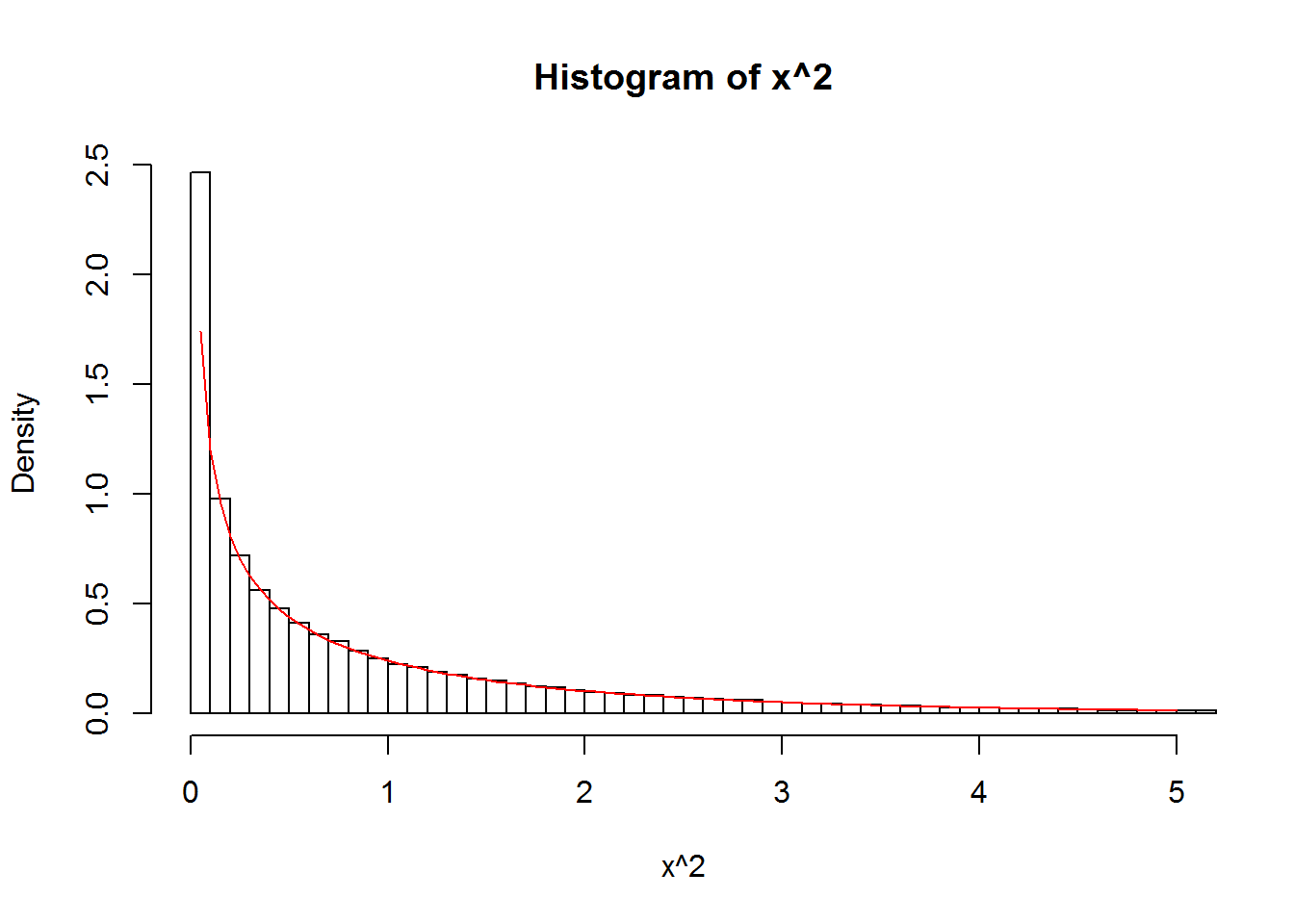Let’s now generate random numbers following the $$\chi^2$$ distribution with df=3 from 3 independent standard normal random variables:

set.seed(2712819)
Q <- rnorm(1e5)^2 + rnorm(1e5)^2 + rnorm(1e5)^2

Note that the above expression is not the same as Q <- 3*rnorm(1e5)^2 since the three sets of random numbers in rnorm(1e5) in the above code chunk are not the same.

Let’s check that Q follows the $$\chi^2$$ distribution with df=3.

hist(Q, freq=FALSE, breaks=100)
curve(dchisq(x,3),col="red",add=TRUE)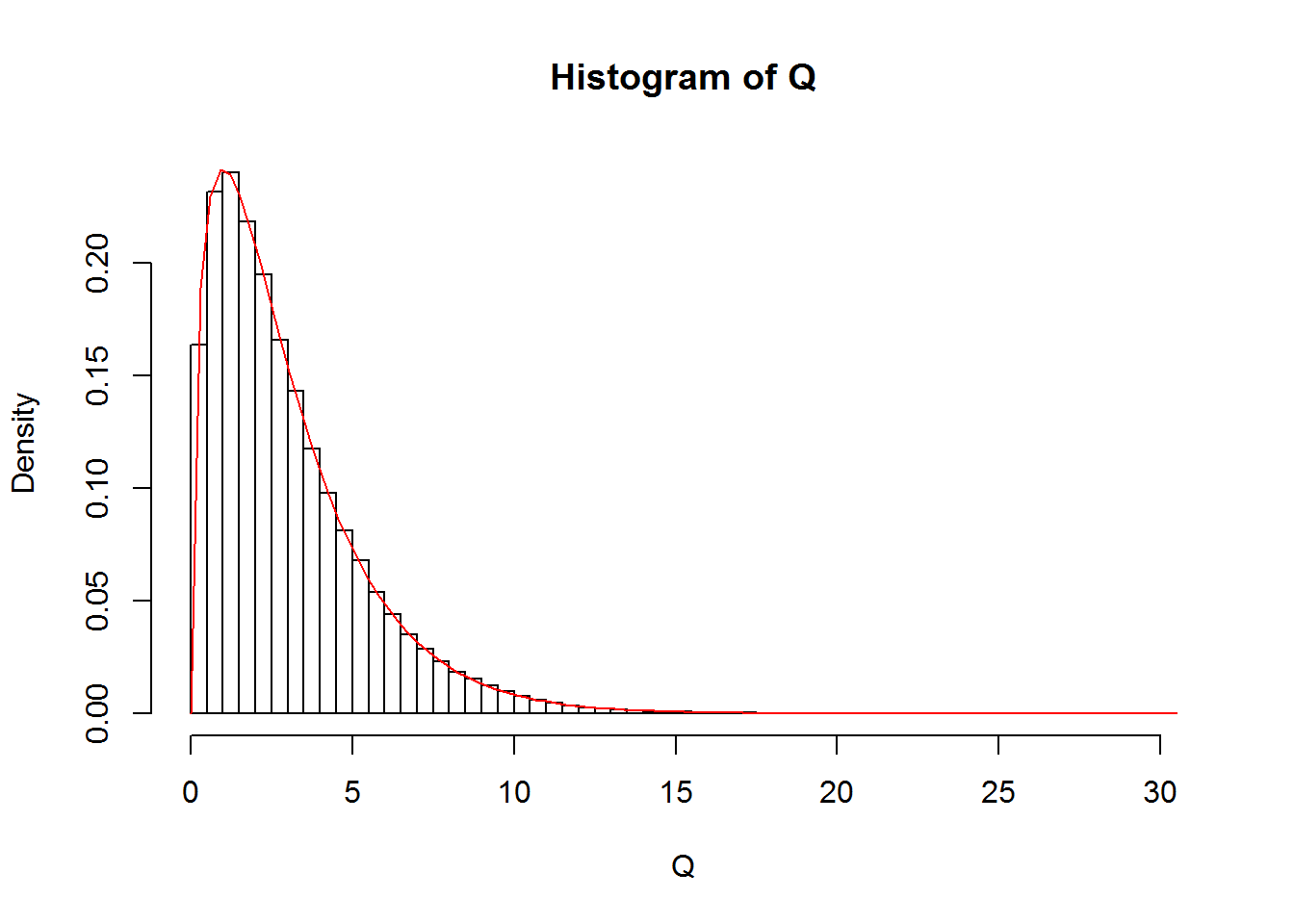In practical applications, we don’t generate random numbers following $$\chi^2$$ distribution from the rnorm() function. The rchisq() function is more convenient to use. For example,

set.seed(90546723)
Q <- rchisq(1e5,10)

generates $$10^5$$ random numbers following the $$\chi^2$$ distribution with 10 degrees of freedom:

hist(Q, freq=FALSE, breaks=100)
curve(dchisq(x,10),col="red",add=TRUE)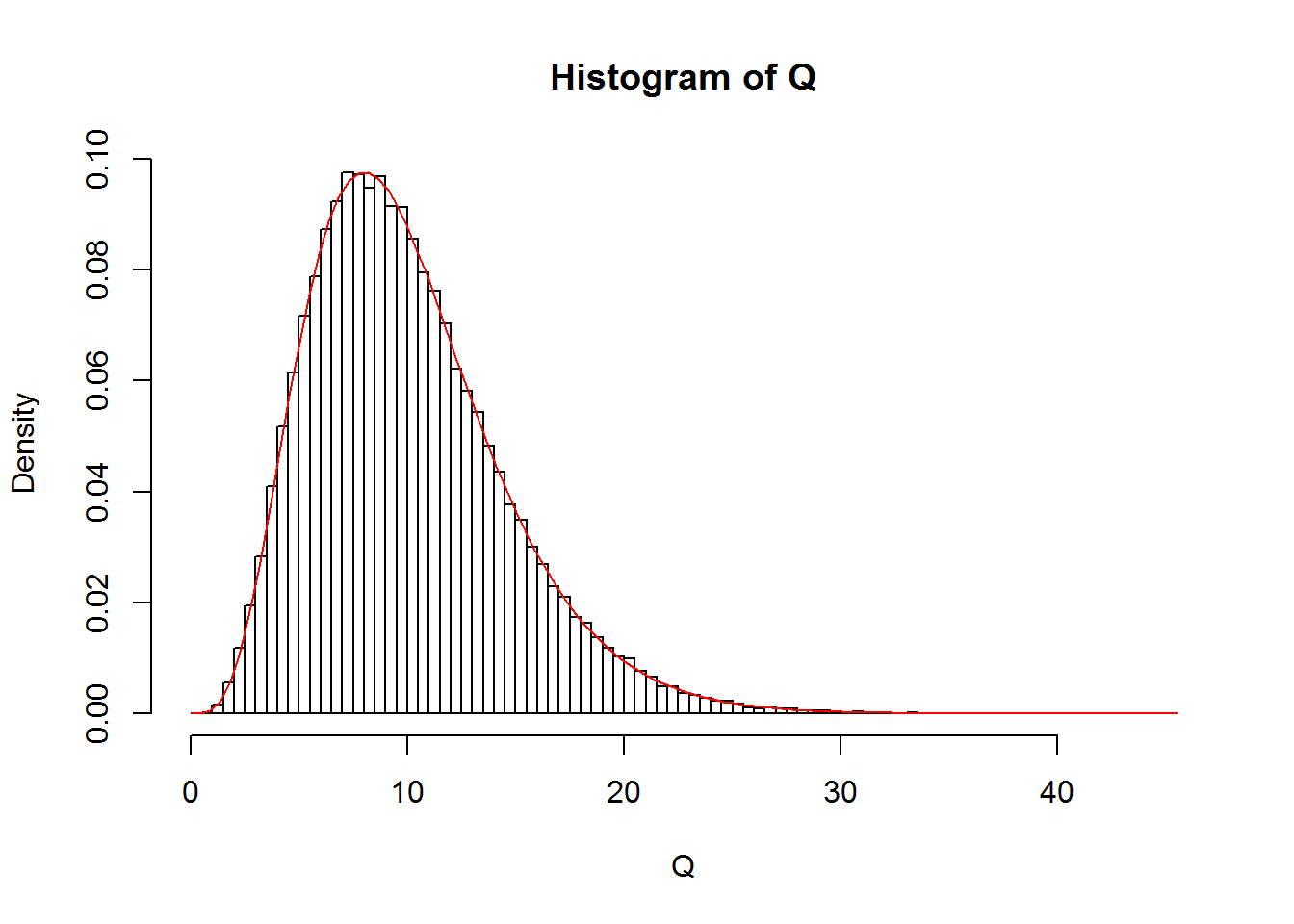### pchisq() and qchisq()

The pchisq(x,df) function returns the cumulative probability $$P(\chi^2<x)$$ for the $$\chi^2$$ distribution with df degrees of freedom, i.e. the area of the shaded region in the plot of the $$\chi^2$$ density curve below.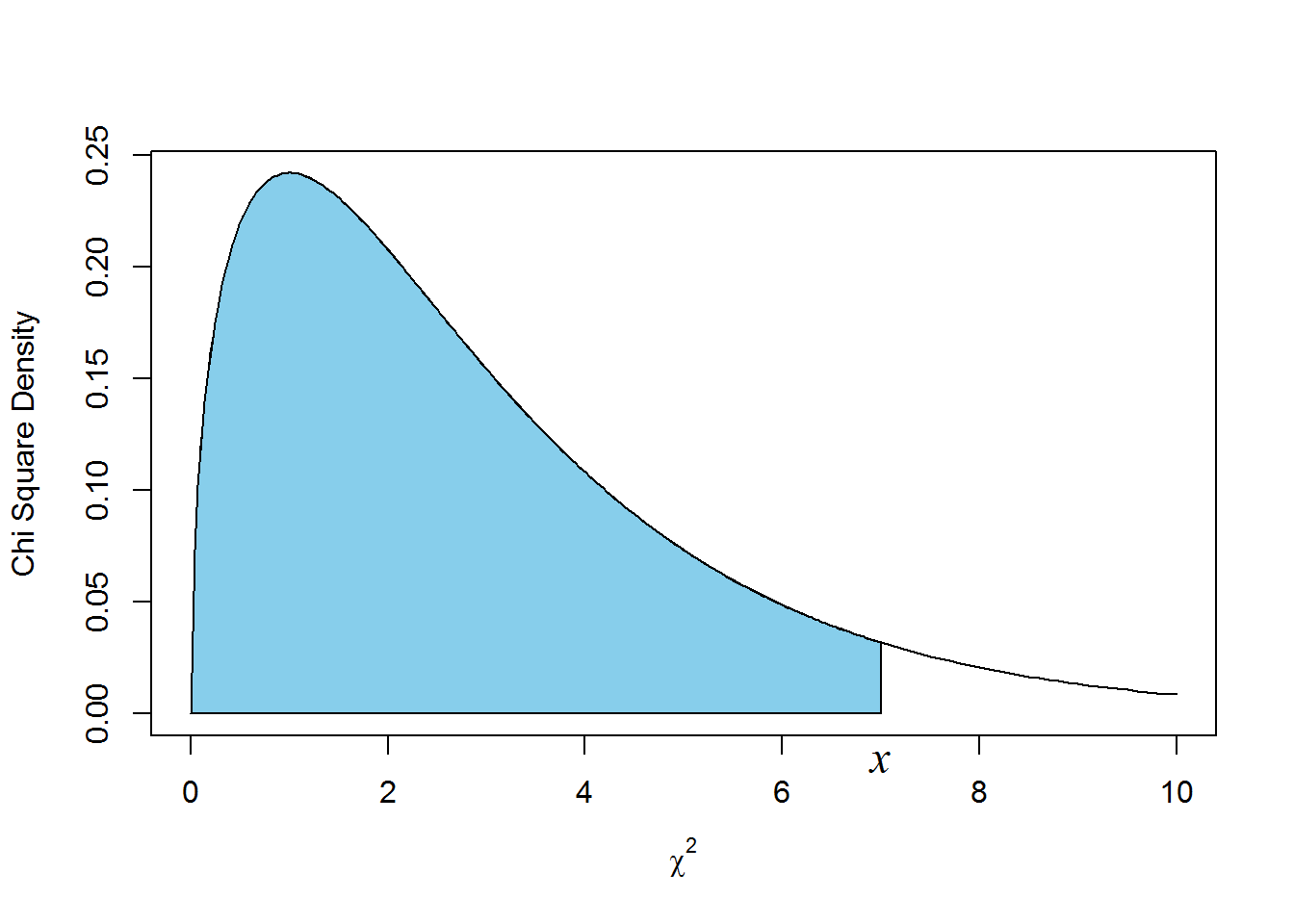For the p-value calculation, we usually want the area of the tail, i.e. $$P(\chi^2>x)$$, the shaded region below.